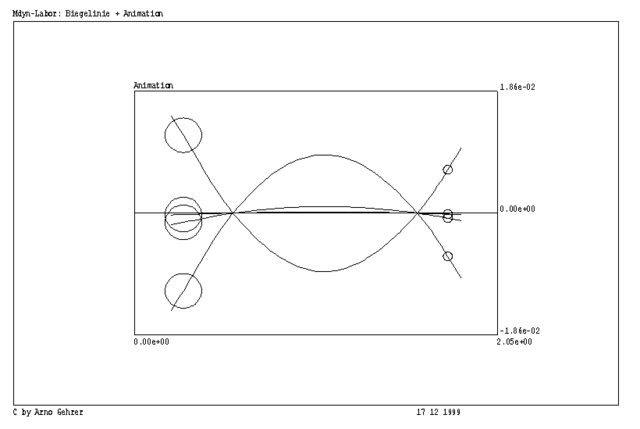# Machine Dynamics

This programm was developed by Arno Gehrer and his diploma student Herbert Reitmayr for the simulation of bending and torsion vibrations.

A computer program for calculating either eigenfrequencies and eigenmodes or enforced vibrations for bending and torsion of rotating shafts has been developed. For the calculation of the bending modes the shafts can be modelled with discrete or continuous mass distribution. The mathematical model representing the discs includes the spinning effect as well as a rotation vertical to the shaft axis. Considering the journal bearings the user can specify four stiffness and four damping coefficients. Regarding the enforced vibrations the rotational speed is varied and the respective results can be visualized graphically by means of computer animation. For the calculation of the torsional vibrations the shafts can also be modelled with discrete or continuous mass distribution. Absorbers can be put in parallel to a torsion spring or connected in series. The results of all calculations are represented by the amplitudes of the quantities related to the respective degrees of freedom and are written to ASCII-files, which can be opened in the Microsoft program Excel.© TTM